# Luna Tech

Tutorials For Dummies.

# 0. 前言

mac 用户可以用 traceroute <website address> 得到详细的 routing 信息。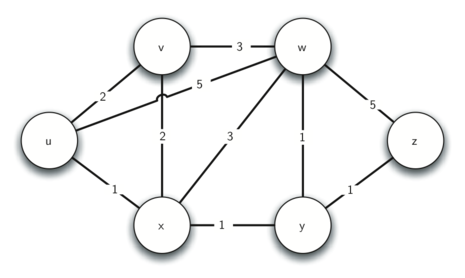# 1. Shortest Path Problems

Word Ladder 里面的 edge 是没有 weight 这个属性的，假如我们这个 routing 的问题中去掉了 weight，就跟 Word Ladder 的问题一模一样~

1. Weighted Graph - Word Ladder
2. Unweighted Graph - Routing

# 2. Dijkstra’s Algorithm

It is important to note that Dijkstra’s algorithm works only when the weights are all positive. You should convince yourself that if you introduced a negative weight on one of the edges to the graph that the algorithm would never exit.

## 逻辑

1. 我们用 dist 这个属性来记录从 starting node 到其他 node 的距离，dist 代表从 starting node 到当前 node 的 total weight。

2. 由于图中的每个 node 都要访问，我们用 priority queue 来决定访问 node 的次序，priority queue 的排列是基于 dist 这个属性的。

3. 我们把每个 node 的 dist 初始值设的非常大，算法结束时每个 node 的 dist 都会成为正确的值。

## 复习

Priority Queue 是基于 Binary Heap 实现的，我们当时实现的那个 PQ 和 Dijkstra 算法 的 PQ 有一些不同。

1. Priority Queue class 存 tuples of key, value pairs, 在 Dijkstra 算法中，Priority Queue 的 key 必须和 node/vertex 的 key 一样；
2. tuple 里面的 value 是用来决定优先级的，我们用 node/vertex 的 distance 作为优先级；
3. 我们有个额外的 method - decreaseKey，当一个 node 已经在 queue 里面时，这个 method 会被调用，来减少 node 的 dist，因为 dist 变少了，所以这个 node 也会被排到优先队列的前面。

## Code

from pythonds.graphs import PriorityQueue, Graph, Vertex
def dijkstra(aGraph,start):
pq = PriorityQueue()
start.setDistance(0)
pq.buildHeap([(v.getDistance(),v) for v in aGraph])
while not pq.isEmpty():
currentVert = pq.delMin()
for nextVert in currentVert.getConnections():
newDist = currentVert.getDistance() \
+ currentVert.getWeight(nextVert)
if newDist < nextVert.getDistance():
nextVert.setDistance( newDist )
nextVert.setPred(currentVert)
pq.decreaseKey(nextVert,newDist)


# 2. 算法图解

## 1. 准备工作

• Starting node: u

• u 有三个邻接 node：v, w, x

• 三个邻接 node 的初始 dist 是 sys.maxint

• 新的 dist 是它们跟 start node 之间的 edge 重量

v: d = 2

w: d = 5

x: d = 1

• 我们把这三个 node 的 predecessor 都设为 u

• dist 是 priority queue 的 value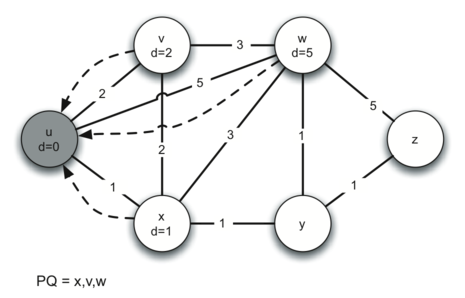## 2. while loop

uv 的距离不需要更新。

w 的距离本来是 5，现在可以减少到 4，我们也更新一下，同时把 w 的 predecessor 改成 x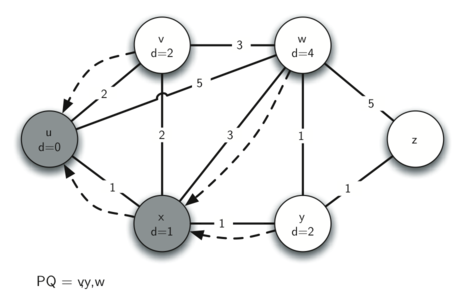## 3. 访问 v

v 的邻接 node 没有什么要改变的 - dist 不需要更新，predecessor 也不需要更新。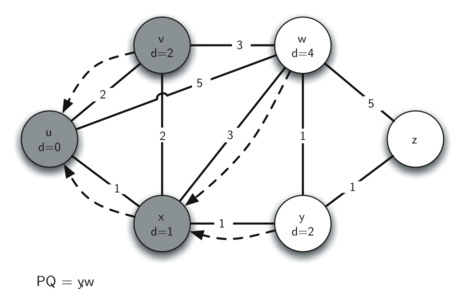## 4. 访问 y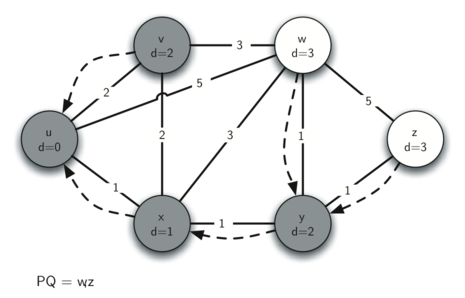## 5. 访问 w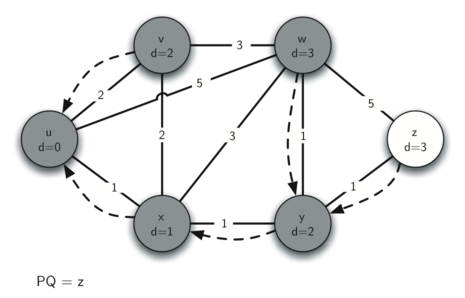## 6. 访问 z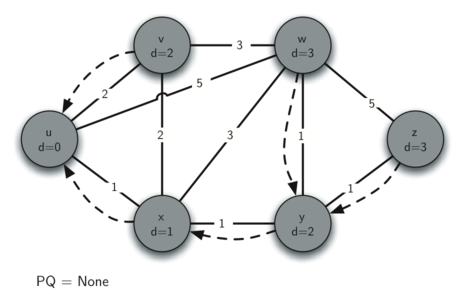# 4. 算法分析

## 局限

1. 不支持负数 weight；
2. 你必须有完整的图才能开始计算；

The implication of this is that every router has a complete map of all the routers in the Internet.

## 复杂度

1. build priority queue - O(V)

每个 node 都访问一次

2. while loop - O(VlogV)

每个 node 都访问一次 - O(V)

在 while loop 里面的 delMin - O(logV)

3. for loop - 𝑂(Elog𝑉)

每个 edge 一次 - O(E)

for loop 里面的 decreaseKey - 𝑂(log𝑉)

O(V)+O(VlogV)+𝑂(Elog𝑉)

= 𝑂((𝑉+𝐸)log(𝑉)).